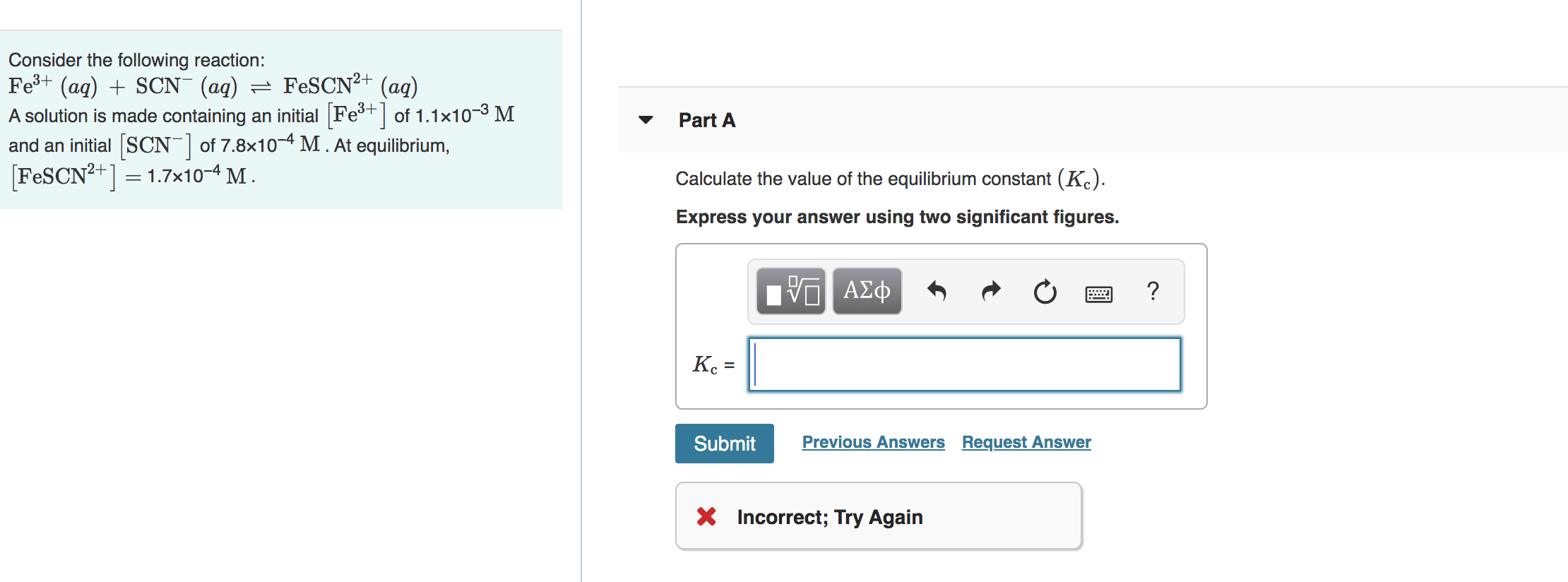# Consider the following reaction Fe^3+(aq) + SCN^-(aq) ⇌ FeSCN^2+(aq) A solution is made containing an initial [Fe^3+] of 1.1×10^-3 M and an initial [SCN^-] of 7.8×10^-4 M. At equilibrium, [FeSCN^2+] = 1.7×10^-4 M. Calculate the equilibrium constant (Kc) Express your answer using two significant figures.